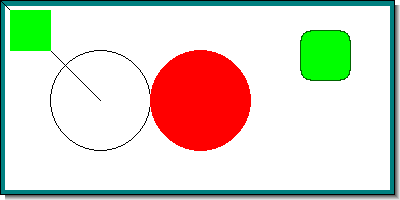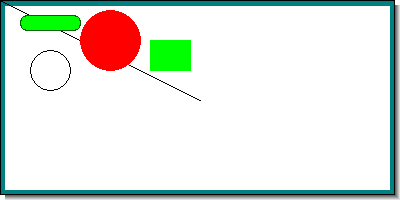## 17.3. Canvas graphs

This is basically a free form blank canvas where any graphics can be drawn. Canvas graphs area also used as the basic graph for graphic tables as described in Chapter 19

The library provides two module files to aid with this "j`pgraph_canvas.php`" and "`jpgraph_canvtools.php`". The second module is strictly speaking not necessary but provides a number of utility classes that makes it much easier to work with canvas.

Canvas graphs is provided as a layer to make is easier to create arbitrary images and still make use of some of the convenience methods available in the library (like font and color support).

Canvas graphs makes it easy to use the low level graphic drawing primitives in the library provided by the two classes

1. `class Image`

This is the basic low level class that encapsulates the GD library by providing a complete framework for using graphic primitives with a coherent API and many convenience methods.

2. `class RotImage`

This is a descendant of class Image that in addition to the basic primitives also provides support for rotation of graphs

It is possible to work at different levels of abstraction when creating a canvas.

1. By only using the basic Image and RotImage classes. This can be considered one abstraction level above the most basic GD libraries

2. By making use of the functionality provided by ```class CanvasGraph``` and the supporting classes (e.g. ```class CanvasScale```). By using the canvas scale class it is possible to defining a coordinate grid on the canvas. These coordinates can then be used instead of absolute pixels which is necessary to us ethe basic Image and RotImage classes.

A concrete example of how canvas graphs can be used can be seen in Figure 8.1 where all the available fonts have been drawn on a canvas.

### 17.3.1. Creating a simple canvas

Creating a canvas gives you the opportunity draw arbitrary shapes on a "white" piece of paper. Let's first show a simple example were we just draw a text box.

Example 17.1. A simple canvas graph to draw a text box (`canvasex01.php`)

 ```1 2 3 4 5 6 7 8 9 10 11 12 13 14 15 16 17 18 19 20 21 22 23 24 25 26 27 28 29 30 31 32 33 34 35 36 ``` ```SetMargin(5,11,6,11); \$g->SetShadow(); \$g->SetMarginColor("teal"); // We need to stroke the plotarea and margin before we add the // text since we otherwise would overwrite the text. \$g->InitFrame(); // Draw a text box in the middle \$txt="This\nis\na TEXT!!!"; \$t = new Text(\$txt,200,10); \$t->SetFont(FF_ARIAL,FS_BOLD,40); // How should the text box interpret the coordinates? \$t->Align('center','top'); // How should the paragraph be aligned? \$t->ParagraphAlign('center'); // Add a box around the text, white fill, black border and gray shadow \$t->SetBox("white","black","gray"); // Stroke the text \$t->Stroke(\$g->img); // Stroke the graph \$g->Stroke(); ?>```

Figure 17.22. A simple canvas graph to draw a text box `(canvasex01.php)`The example in Figure 17.22 starts by creating a 400x200 sized image.

The margins are then set to to get a frame around the image.

For canvases the margins has no effect in the way coordinates are entered. Top left is (0,0) and bottom right (including any potential margin and shadow) is the maximum. In this case the coordinate range are X:0-399, and Y:0-199

The `InitFrame()` method is then called which actually strokes the margin and plotarea to the graph. Since everything is stroked in the order the commands are given it is necessary to make sure that the graphical objects that should be on top are stroked last. This is different from the other graph types since they will hide these details and stroke the details in the graph in the correct order.

We then create a Text object, setup it's properties, including the absolute screen position where we want the text, and then stroke it.

The `Text::Align()` method specifies the anchor point of the text as explained in Chapter 8

We also specify that the lines within the paragraph should be centered with a call to `Text::ParagraphAlign()` Since we also choose to have a box around the text we have to make use of the method `Text::SetBox()` which is used to specify the fill color, the border color and the shadow color (if the shadow color is set to '', no shadow will be used).

Now we are ready to stroke the text onto the canvas. In order to do so we must specify the graphical context when stroking the text. The current graphic context is always available in the "`\$img`" property of the CanvasGraph class.

This specification of the graphical context is needed to all Stroke method that is external to the main graph class.

### 17.3.2. Adding lines and rectangles to a canvas

A canvas also makes a good background for using standard graphic primitives, for example circles and lines. The first thing to remember is that this requires working with absolute screen coordinates and secondly that all drawing primitives are found in the Image Class accessible as a property of the Graph class.

This means that to draw a line between absolute coordinates (0,0) and (100,100) the following code must be created

 ```1 ``` `\$graph->img->Line(0, 0, 100, 100);`

The following example shows how to create some basic graphic shapes on a canvas

Example 17.2. Drawing some basic geometric shapes on a canvas (`canvasex02.php`)

 ```1 2 3 4 5 6 7 8 9 10 11 12 13 14 15 16 17 18 19 20 21 22 23 24 25 26 27 28 29 30 31 32 33 34 35 36 37 38 39 40 41 ``` ```SetMargin(5,11,6,11); \$g->SetShadow(); \$g->SetMarginColor("teal"); // We need to stroke the plotarea and margin before we add the // text since we otherwise would overwrite the text. \$g->InitFrame(); // Add a black line \$g->img->SetColor('black'); \$g->img->Line(0,0,100,100); // .. and a circle (x,y,diameter) \$g->img->Circle(100,100,50); // .. and a filled circle (x,y,diameter) \$g->img->SetColor('red'); \$g->img->FilledCircle(200,100,50); // .. add a rectangle \$g->img->SetColor('green'); \$g->img->FilledRectangle(10,10,50,50); // .. add a filled rounded rectangle \$g->img->SetColor('green'); \$g->img->FilledRoundedRectangle(300,30,350,80,10); // .. with a darker border \$g->img->SetColor('darkgreen'); \$g->img->RoundedRectangle(300,30,350,80,10); // Stroke the graph \$g->Stroke(); ?>```

Figure 17.23. Drawing some basic geometric shapes on a canvas `(canvasex02.php)`### 17.3.3. Using a canvas scale together with the shape class

The previous method using absolute coordinates works. But it gives very little flexibility in, for example, scaling the image. Working with absolute coordinates is therefore not very practical and gets tedious for complex figures.

To mitigate these issues the library offers a convenience class, ```class CanvasScale```, this will allow the creation of a suitable scale that will make it easier to both create and scale the graph.

In order to use a canvas scale the module "`jpgraph_canvtools.php`" must first be included. In addition to the canvas scale class this module also provides the companion `Shape` class. This companion shape class is used to draw shapes using the specified scale onto the canvas.

Using the scale is quite simple and requires only two steps

1. An instance of the scale class is first created with the CanvasGraph instance as the first parameter

2. The wanted scale is then specified with the wanted x and y scales

The following code snippet shows how this is done

 ```1 2 3 4 5 6 7 8 9 10 11 ``` ```Set(\$xmin, \$xmax, \$ymin, \$ymax); // ... ?>```

The next step needed to be able to create geometric shapes is to create an instance of class Shape and give the canvas graph and the scale as the two parameters as the following line shows.

 ```1 2 3 ``` ``````

It is now possible to create arbitrary geometric shapes by using the provided methods in the shape class. The shape class provides the following methods

• `Shape::SetColor(\$aColor)`

• `Shape::Line(\$x1,\$y1,\$x2,\$y2)`

• `Shape::Polygon(\$p,\$aClosed=false)`

• `Shape::FilledPolygon(\$p)`

• `Shape::Bezier(\$p,\$aSteps=40)`

• `Shape::Rectangle(\$x1,\$y1,\$x2,\$y2)`

• `Shape::FilledRectangle(\$x1,\$y1,\$x2,\$y2)`

• `Shape::Circle(\$x1,\$y1,\$r)`

• `Shape::FilledCircle(\$x1,\$y1,\$r)`

• `Shape::RoundedRectangle(\$x1,\$y1,\$x2,\$y2,\$r=null)`

• `Shape::FilledRoundedRectangle(\$x1,\$y1,\$x2,\$y2,\$r=null)`

• `Shape::ShadowRectangle(\$x1,\$y1,\$x2,\$y2,\$fcolor=false,\$shadow_width=null,\$shadow_color=array(102,102,102))`

• `Shape::SetTextAlign(\$halign,\$valign="bottom")`

• `Shape::StrokeText(\$x1,\$y1,\$txt,\$dir=0,\$paragraph_align="left")`

• ```Shape:: IndentedRectangle(\$xt,\$yt,\$w,\$h,\$iw=0,\$ih=0,\$aCorner=3,\$aFillColor="",\$r=4)```

We can now show a complete example of using the shape class

Example 17.3. Creating a canvas graph with a scale and using the shape class (`canvasex03.php`)

 ```1 2 3 4 5 6 7 8 9 10 11 12 13 14 15 16 17 18 19 20 21 22 23 24 25 26 27 28 29 30 31 32 33 34 35 36 37 38 39 40 41 42 43 44 45 46 47 48 49 50 51 52 53 54 55 56 57 ``` ```SetMargin(5,11,6,11); \$g->SetShadow(); \$g->SetMarginColor("teal"); // We need to stroke the plotarea and margin before we add the // text since we otherwise would overwrite the text. \$g->InitFrame(); // Create a new scale \$scale = new CanvasScale(\$g); \$scale->Set(0,\$xmax,0,\$ymax); // The shape class is wrapper around the Imgae class which translates // the coordinates for us \$shape = new Shape(\$g,\$scale); \$shape->SetColor('black'); // Add a black line \$shape->SetColor('black'); \$shape->Line(0,0,20,20); // .. and a circle (x,y,diameter) \$shape->Circle(5,14,2); // .. and a filled circle (x,y,diameter) \$shape->SetColor('red'); \$shape->FilledCircle(11,8,3); // .. add a rectangle \$shape->SetColor('green'); \$shape->FilledRectangle(15,8,19,14); // .. add a filled rounded rectangle \$shape->SetColor('green'); \$shape->FilledRoundedRectangle(2,3,8,6); // .. with a darker border \$shape->SetColor('darkgreen'); \$shape->RoundedRectangle(2,3,8,6); // Stroke the graph \$g->Stroke(); ?>```

Figure 17.24. Creating a canvas graph with a scale and using the shape class `(canvasex03.php)`To show the usefulness of using the scale class Figure 17.25 shows the result after just changing the image size but keeping the code the same. The relation between the shapes will remain the same but in a smaller format to fit the new image size.

Figure 17.25. Changing the image size to create a smaller version of the previous example `(canvasex04.php)`If we instead wanted to keep the image size but shrink the shapes we could just make the scale twice as large which would result in Figure 17.26

Figure 17.26. Keeping the image size but changing the scale `(canvasex05.php)`We previously mentioned that the Shape class was a wrapper around the image class with one exception. The exception is that it contains one additional method which draws an "indented rectangle". An indented rectangle is a rectangle where one of it's four corners have been moved into the rectangle Figure 17.27 shows an example of using this shape.

Figure 17.27. Example of using an indented rectangle `(canvasex06.php)`As a final note we mention the utility class ```class CanvasRectangleText``` This can be used to add a text with a rounded rectangle (possibly filled) onto the canvas. Figure 8.1 where all the available fonts were shown were using this class.